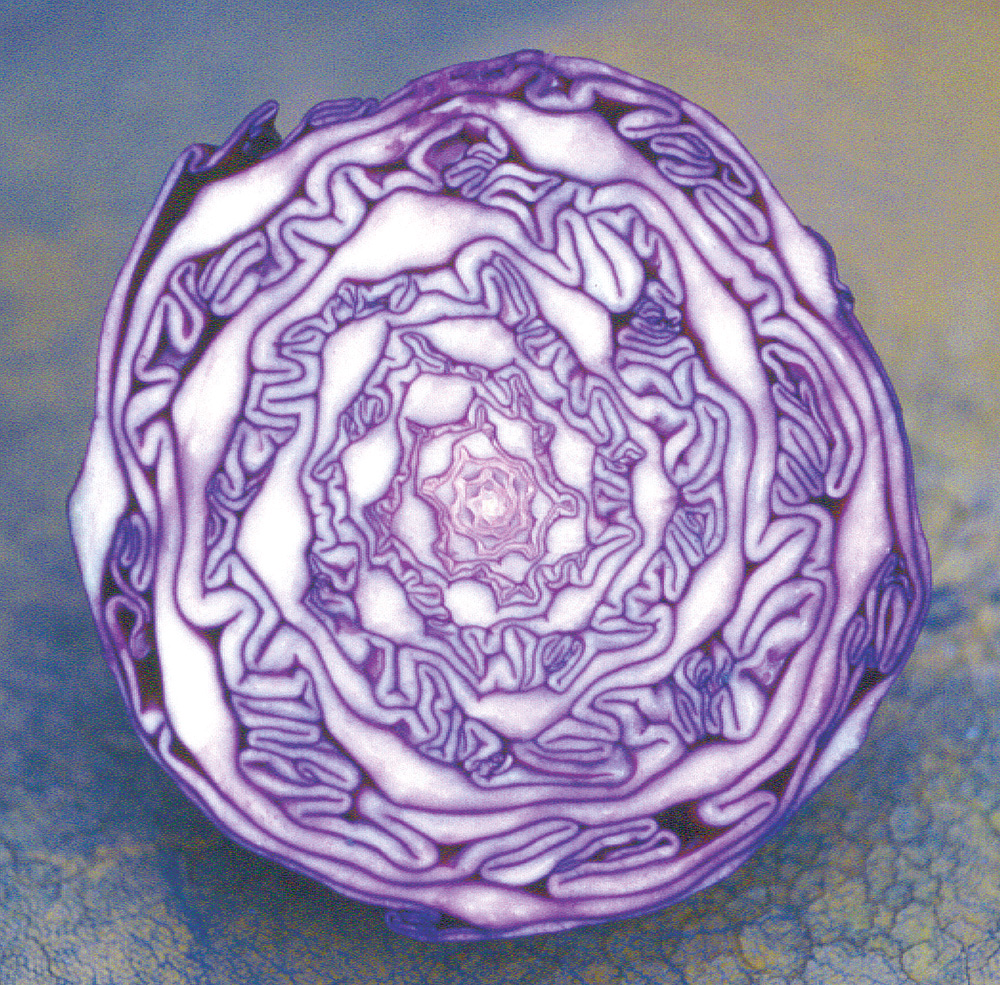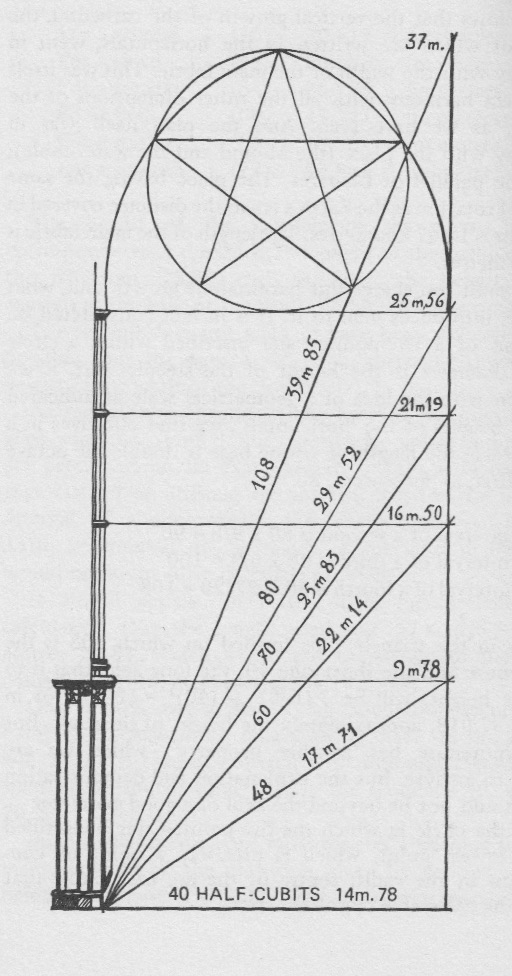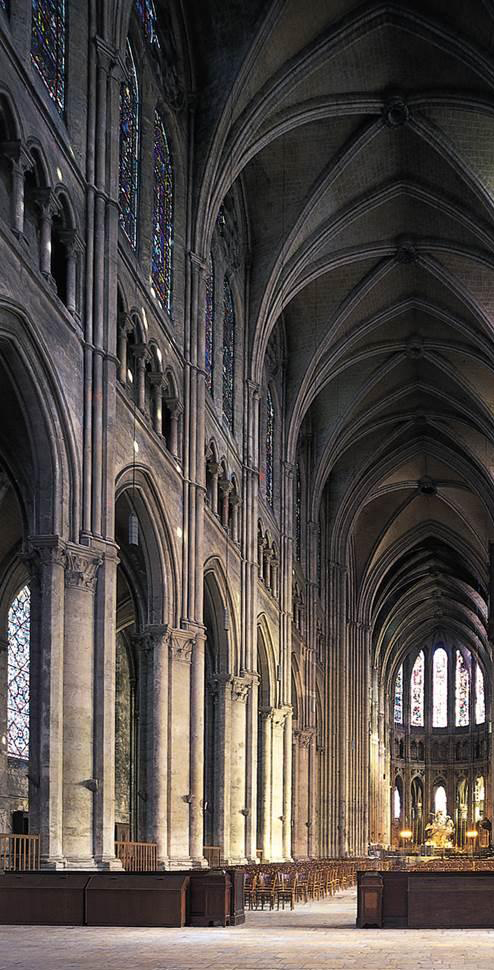## Proportion

We may regard the phenomenal world, or nature, and music as two different expressions of the same thing.

Simple proportional relationships underlie both music and physical pattern-forming processes. We have seen that Nature shows a preference for simple number ratios in sonic structuring. By analogy, simple geometric forms and proportions are the archetypes for structural development in the material world. What we hear and what we see appear to share common principles and evolve in similiarly proportional ways. Just as there are scalar reflections of tone in rhythm, so there are echoes of proportional spirals on the cosmic scale in galaxies as well as on a more mundane level in the cross-section patterning of a red cabbage (figure 48).Figure 48

Harmonic researchers of the last century, such as Hans Kayser and Rudolf Haase, have sought to reach beyond this vague analogy and consider the musical and the physical as direct reflections of each other, sharing at their most basic vibrational level an identity of nature rather than incidental similarities . If, as Pythagoras believed, a stone is frozen music, then we should be able to trace some kindred geometry of vibration.Figure 49

An angle, like a ratio, can be thought of as a relationship between two numbers. Figure 49 shows the musical ratios of the harmonic canon transposed as angles of declination. These angles are the product of an x and a y vector, corresponding with the over and under number of a musical ratio. So in figure 50, 1/1 when considered as the base and vertical of a right-angled triangle, produces an internal angle of 45 degrees. The angled vectors of less than 45 degrees in figure 50 represent musical ratios which are higher than 1/1 in pitch while lower tones have a correspondingly steeper angle.Figure 50

Goethe’s dictum that architecture is frozen music has a clear antecedent in Gothic cathedrals. Medieval master masons used musical proportions as an integral part of their designs. Consider the angles of elevation in Chartres cathedral (figure 51).Figure 51

The horizontal lines are clearly delineated in the elevation plan; and we can see that the ratios between the hypoteneuse and the adjacent lengths as shown on the left are musically harmonious. So 48/40 half cubits equates to the ratio 6/5 (a minor third); 60/40 equates to 3/2 (a fifth); 70/40 to 7/4 (a natural seventh) and 80/40 to 2/1 (an octave). 108/40 is a bit more problematic - 27/20 (a rather flat fourth).

Let’s pursue this musical approach by examining the angles and relative lengths inherent within a hexagon.

Hexagonal patterning is widespread in nature from honeycombs to the molecular structure of the graphite of the geometer’s pencil. Nature favours this geometric archetype because of its capacity for space-filling; efficiency is always paramount in nature’s decisive processes. Can this form then be transposed into the musical realm?Figure 52

When a right-angled triangle is inscribed within a hexagon (figure 52), its three sides constitute the possible internal dimensions of the larger form. The baseline is both the length of the hexagon’s side and the radius of the enclosing circle while the hypotenuse is the diameter of this circle, therefore twice the length of the baseline. Musical problems arise, however, when we consider the remaining vertical side. The ratio of the baseline to the vertical is 1/√ 3, hardly the most consonant of musical ratios, since consonance derives from the relationship of simple whole numbers, not irrational ones. The full extent of this impasse between musical ratios and geometric form can be gauged by tabulating the musical/geometric features of a variety of right-angled triangles which derive from either musical consonance or the simplicity of spatial form (figure 53).Figure 53

In this table, a signifies the baseline, b the vertical and c the hypotenuse. The resultant angle between the baseline and the hypotenuse is shown in the x column while the musical ratio of the baseline to the vertical appears in the final column. There is a clear incompatiblity in this musical geometry: the angle and lengths which derive from the simple geometry of the hexagon (line 3) are musically dissonant, while consonant ratios (lines 2, 4 and 5) produce angles which are inimicable to geometric development.

The major stumbling block in any attempt to link music and the patterns of nature is the omnipresence of irrational numbers in geometric structuring. Square roots, for instance, cannot be comprehended musically - a realization which was not lost on Pythagoras, who ordered one of his followers, Hippasus, to be drowned at sea for revealing the musical irrationality of !2.

In particular, the irrational Golden Proportion (f, phi), appears impervious to musical equivalence.

If a ratio is a comparison between two values, then, strictly speaking, a proportion is a comparison between two ratios. Now the Golden Proportion is the simplest proportion in terms of its form, since it is the only one which can be expressed with only two terms ( a : b :: b : [a+b] ). Due to this proportional simplicity it is ubiquitous in natural processes of growth from the galaxy and cabbage of figure 48 to the idealized proportions of the human body.

The connection between this proportion and the Fibonacci series has been well documented. In this series each term is the sum of the previous two (1, 2, 3, 5, 8, 13.....). If we procede by dividing each term by its predecessor, a distinct ratio progression emerges which tends towards the irrational Golden Proportion - 2/1, 3/2, 5/3, 8/5, 13/8 etc.. Each successive ratio is a closer approximation to 1.618.., the major part of the Golden Section, while the inversion of the series (1/2, 2/3, etc.) approximates the minor part, 0.618 (figure 54).Figure 54

These two ratio series can be considered musically and transposed into the Lambdoma matrix (figure 55). Hans Kayser, amongst others, has thereby viewed these radiating progressions as direct musical reflections of the geometric Golden Proportion. However, the problem cannot be so easily solved. As these progressions procede downwards through the Lambdoma they do indeed mirror the Golden Proportion with increasing refinement. But coupled with this refinement is a process of ever increasing dissonance. The musical relationship between the Golden Proportion and unity is infinitely dissonant, not the most promising of musical/geometric correspondances.

Figure 55 (Click to Play)

Is it possible, then, to build a bridge between musical consonance and the archetypal geometry of nature, or is the issue as intractable as the music/colour debate which I dwelt on earlier?

Hazrat Inayat Khan, the Sufi mystic whom I quoted initially said that “all we see in this objective world, every form, has been constructed by sound and is the phenomenon of sound.” This is no esoteric flight of fancy but a belief well-founded on empirical evidence. There may not be a simple one to one correspondance between tonal ratios and material form, but the action of musical tone on physical matter cannot be denied. For when elastic physical substances are excited by tonal vibration they display geometric symmetries akin to patterns which recur throughout nature.

How these patterns emerge and interpenetrate under the influence of harmonic tone is a major objective of this research.

Cymatics